Next: Jacobi Ellipsoids Up: Hydrostatics Previous: Equilibrium of a Rotating

# Maclaurin Spheroids

One, fairly obvious, way in which the constraint (2.115) can be satisfied is if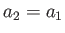. In other words, if the planet is rotationally symmetric about its axis of rotation. An ellipsoid that is rotationally symmetric about a principal axis--or, equivalently, an ellipsoid with two equal principal radii--is known as a spheroid. In fact, ifthen the cross-section of the planet's outer boundary in any plane passing though the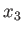-axis is an ellipse of major radius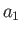in the direction perpendicular to the-axis, and minor radius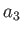in the direction parallel to the-axis. Here, we are assuming that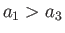: that is, the planet is flattened along its axis of rotation. The degree of flattening is conveniently measured by the eccentricity,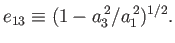(2.118)

Thus, if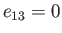then there is no flattening, and the planet is consequently spherical, whereas if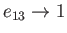then the flattening is complete, and the planet consequently collapses to a disk in the-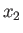plane.

Let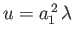and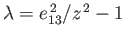. Settingin Equation (2.114), we obtain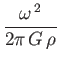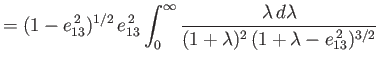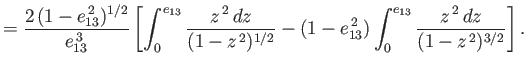(2.119)

Performing the integrals, which are standard (Speigel, Liu, and Lipschutz 1999), we find that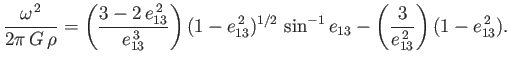(2.120)

This famous result was first obtained by Colin Maclaurin (1698-1746) in 1742. Finally, in order to calculate the potential energy, (2.116), we need to evaluate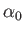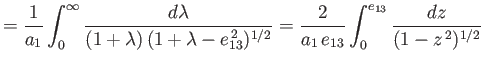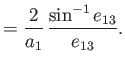(2.121)

Table 2.1: Properties of the Maclaurin spheroids.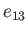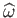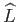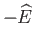0.00 0.00000 0.00000 0.60000 0.60 0.31729 0.18037 0.56233 0.05 0.02582 0.01266 0.59980 0.65 0.34484 0.20286 0.55320 0.10 0.05168 0.02540 0.59919 0.70 0.37239 0.22834 0.54200 0.15 0.07758 0.03830 0.59817 0.75 0.39967 0.25792 0.52800 0.20 0.10357 0.05144 0.59672 0.80 0.42612 0.29345 0.51001 0.25 0.12967 0.06491 0.59479 0.85 0.45046 0.33833 0.48587 0.30 0.15591 0.07882 0.59236 0.90 0.46932 0.39994 0.45107 0.35 0.18231 0.09329 0.58936 0.95 0.47045 0.50074 0.39272 0.40 0.20889 0.10846 0.58572 0.96 0.46472 0.53194 0.37485 0.45 0.23567 0.12450 0.58135 0.97 0.45418 0.57123 0.35273 0.50 0.26267 0.14163 0.57612 0.98 0.43475 0.62486 0.32351 0.55 0.28989 0.16013 0.56986 0.99 0.39389 0.71209 0.27916

Let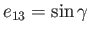. Thus,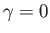corresponds to no rotational flattening, andto complete flattening. Moreover,and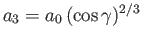, where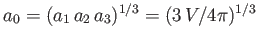is the mean radius. It is also helpful to define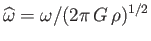,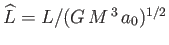, and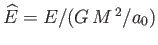. The previous analysis leads to the following set of equations that specify the properties of the so-called Maclaurin spheroids: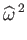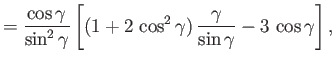(2.122)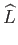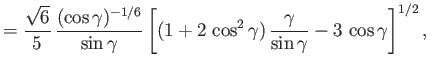(2.123)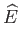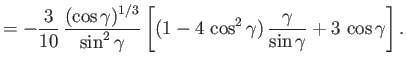(2.124)

These properties are set out in Table 2.1.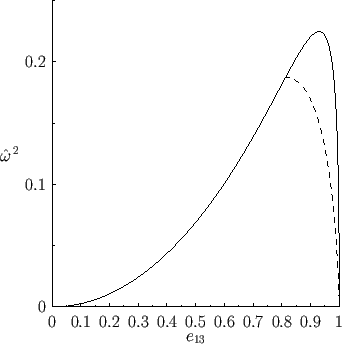In the limit,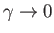, in which the planet is relatively slowly rotating (i.e.,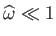), and its degree of flattening consequently slight, Equations (2.122)-(2.124) reduce to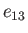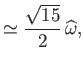(2.125)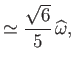(2.126)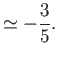(2.127)

In other words, in the limit of relatively slow rotation, when the planet is almost spherical, its eccentricity becomes directly proportional to its angular velocity. In this case, it is more conventional to parameterize angular velocity in terms of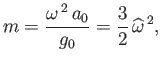(2.128)

where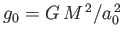is the mean surface gravitational acceleration. Furthermore, the degree of rotational flattening is more conveniently expressed in terms of the ellipticity,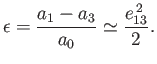(2.129)

Thus, it follows from (2.125) that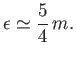(2.130)

For the case of the Earth (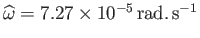,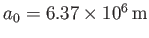,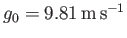--Yoder 1995), we obtain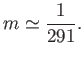(2.131)

Thus, it follows that, were the Earth homogeneous, its figure would be a spheroid, flattened at the poles, of ellipticity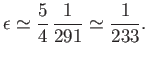(2.132)

This result was first obtained by Newton. The actual ellipticity of the Earth is about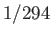(Yoder 1995), which is substantially smaller than Newton's prediction. The discrepancy is due to the fact that the Earth is strongly inhomogeneous, being much denser at its core than in its outer regions.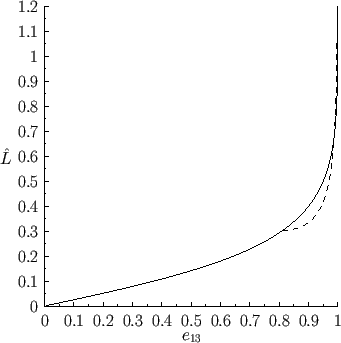Figures 2.5 and 2.6 illustrate the variation of the normalized angular velocity,, and angular momentum,, of a Maclaurin spheroid with its eccentricity,, as predicted by Equations (2.122)-(2.124). It can be seen, from Figure 2.5, that there is a limit to how large the normalized angular velocity of such a spheroid can become. The limiting value corresponds to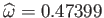, and occurs when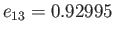. For values oflying below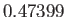there are two possible Maclaurin spheroids, one with an eccentricity less than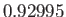, and one with an eccentricity greater than. Note, however, from Figure 2.6, that despite the fact that the angular velocity,, of a Maclaurin spheroid varies in a non-monotonic manner with the eccentricity,, the angular momentum,, increases monotonically with, becoming infinite in the limit. It follows that there is no upper limit to the angular momentum of a Maclaurin spheroid.Next: Jacobi Ellipsoids Up: Hydrostatics Previous: Equilibrium of a Rotating
Richard Fitzpatrick 2016-03-31